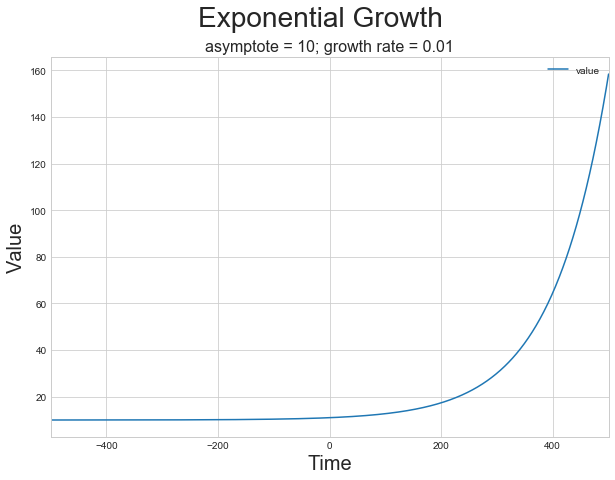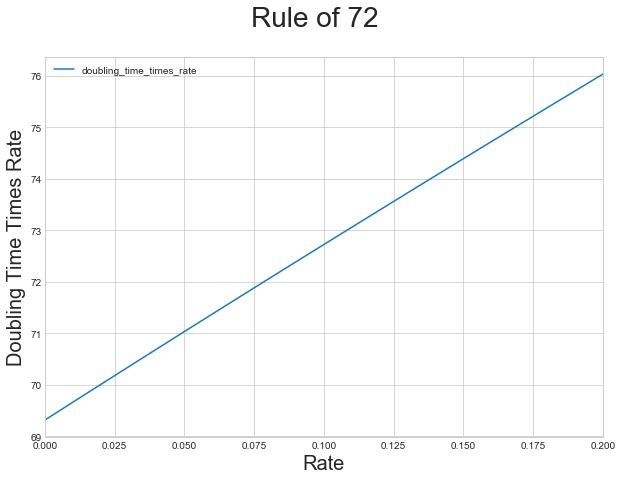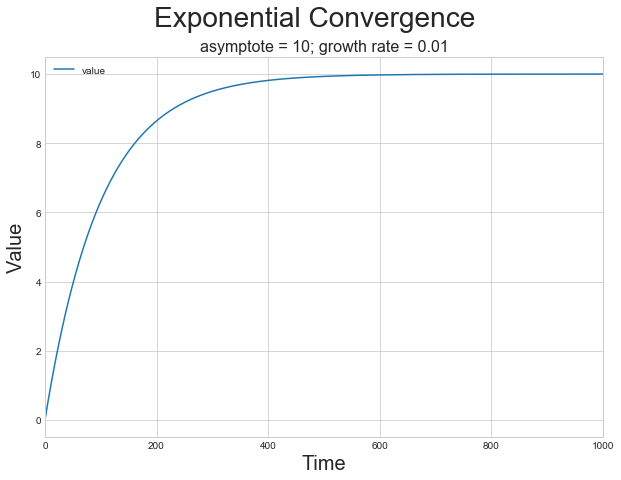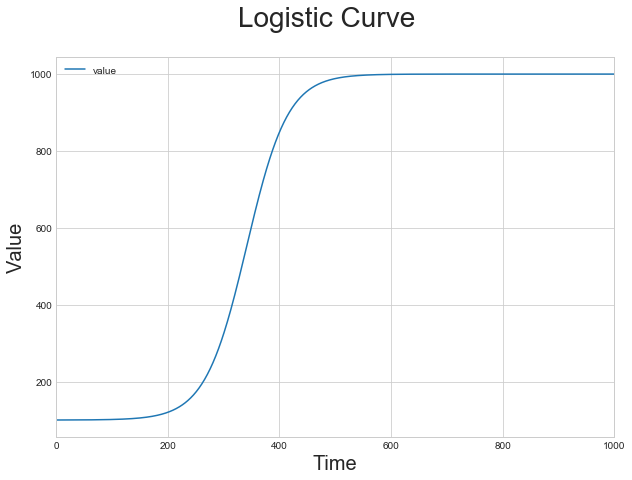## Analyzing Growth: Lecture Support for Econ 113 S2018

I am going to want my American Economic History students this semester to be able to handle three sets of economic tools:

1. Supply and demand, with the calculation of equilibrium prices and quantities, consumer and producer surplus, tax (and other) wedges, and deadweight losses

2. Keynesian national income accounting/monetarist quantity theory, for depressions and inflationary gaps

3. Compound growth, with doubling and thousand-fold times for constant compounding rates

So I will park the calculations file for the lecture of (3) here for convenience...

# 2018-02-04 Econ 113 Analyzing Growth¶

## Setting up the Python/Jupyter environment¶

In :
%%javascript

IPython.OutputArea.prototype._should_scroll = function(lines) {
return false;}

In :
# keep output cells from shifting to autoscroll: little scrolling
# subwindows within the notebook are an annoyance...


# Tools: Analyzing Growth¶

• Economics gives us numbers: prices and quantities over time
• Proportional growth—compound interest
• The time over which some growth process takes place
• The rate at which growth (or shrinkage) takes place
• The amount that the variable grows to
• T, g, y… with little t standing in for any potential moment
• Don’t get snowed! Accurately assess how important or consequential things are!

## The Usefulness of Math¶

Listen to Richard Feynman:To make calculations, the Maya had invented a
system of bars and dots to represent numbers…
rules… to calculate and predict… the risings
and settings of Venus…. Only a few Maya priests
could do such elaborate calculations….

Suppose we were to ask one of them how to do
just one step in the process of predicting when
Venus will next rise as a morning star—subtracting
two numbers….. How would the priest explain?….

He could either teach us the… bars and dots and
the rule… or he could tell us what he was really doing:

Suppose we want to subtract 236 from 584.
First, count out 584 beans and put them in
a pot. Then take out 236 beans and put them
to one side. Finally, count the beans left
in the pot. That number is the result…

You might say, ‘My Quetzalcoatl! What
tedium… what a job!’

To which the priest would reply:

That’s why we have the rules…. The rules
are tricky, but they are a much more efficient
way of getting the answer…. We can predict
the appearance of Venus by counting beans
(which is slow, but easy to understand) or
by using the tricky rules (which is much
faster, but you must spend years in school to learn them)…Muḥammad ibn Mūsā al-Khwārizmī (c. 780-850):

• Al-Kitāb al-Mukhtaṣar fī Hisāb al-Jabr wa’l-Muḳābala
• The Compendious Book on Calculation by
Restoration and Balancing
• Worked in Baghdad at the House of Wisdom established by the Kalif Al-Mamun
• Why "al_Khwārizmī"?
• Muhammed = "the praiseworthy, the glorified"
• Mose = "is born" (Thutmose = "Thoth is born")
• How many Muhammed ibn Mūsās were wandering
• Algorithm...Isaac Newton (1642-1727):

• Philosophiæ Naturalis Principia Mathematica
• Mathematical Principles of Natural Philosophy
• Worked in Cambridge at the university there
• Except when he fled the plague...
• Arithmetic and accounting
• Algebra and calculus
• What-if machines—ways of doing a huge number
of potential calculations all at once…

## Exponential Growth¶

$\frac{dy}{dt} = g(y - a)$

• g = 0.01
• a = 10
• Start at t = -500 with y = a + 0.00674
• Does nothing for a long time—stays very near 10—
then explodes
• and keeps on exploding...
• Let's see:
In :
# make sure graphs are displayed in main notebook
%matplotlib inline

import numpy as np                 # make sure libraries are
import pandas as pd                #  in the namespace
import matplotlib as mpl
import matplotlib.pyplot as plt
from IPython.display import Image

plt.style.use('seaborn-whitegrid') # graphics setup
figure_size = plt.rcParams["figure.figsize"]
figure_size = 10
figure_size = 7
plt.rcParams["figure.figsize"] = figure_size

a = 10                             # set the initial asymptote
g = 0.01                           # set the growth rate
array = np.zeros((1001,2))         # initialize an array
exponential_function_df = pd.DataFrame(array,
columns=["time","value"])      # create a dataframe

# calculate values for the exponential function

for t in range(-500,501, 1):
exponential_function_df.time[t+500] = t
exponential_function_df.value[t+500] =  a + np.exp(g*t)

exponential_function_df = exponential_function_df.set_index("time")
exponential_function_df.plot()     # and graph

plt.ylabel("Value", fontsize=20)   # set labels
plt.xlabel("Time", fontsize=20)
plt.suptitle("Exponential Growth", fontsize=28)
plt.title("asymptote = 10; growth rate = 0.01", fontsize=16)

Out:
<matplotlib.text.Text at 0x1160fb0b8>Math presentation can be ugly inline:

• dy/dt = g(y - a)
• y = 10 + exp(0.01(t-10))

Or prettier:

(1)           $\frac{dy}{dt} = d(y - a)$

(2)           $y = 10 + e^{0.01(t-10}$

Rule of 72 for Exponential Growth:

• Rules of thumb for a growth rate g:
• (y-a) compounded continuously doubles every 0.693/g periods
• (y-a) grows a thousandfold every 6.91/g periods
• But we use the Rule of 72:
• Why? 72 has many divisors
• Why? 69.3—ln(2)—is for continuous compounding
• Let's see:
• Why is the thousandfold time ten times the doubling time?
• 1024 = 2^10
• ln(1024) = 10 * ln(2)
In :
array = np.zeros((21,2))         # initialize an array
rule_of_72_df = pd.DataFrame(array,
columns=["rate","doubling_time_times_rate"])      # create a dataframe

rule_of_72_df.rate = 0
rule_of_72_df.doubling_time_times_rate = np.log(2)*100

for i in range(1, 21):
rule_of_72_df.rate[i] = i*.01
rule_of_72_df.doubling_time_times_rate[i] = (i *
np.log(2)/np.log(1 + i*.01))

rule_of_72_df = rule_of_72_df.set_index("rate")
rule_of_72_df.plot()
plt.ylabel("Doubling Time Times Rate", fontsize=20)   # set labels
plt.xlabel("Rate", fontsize=20)
plt.xlim(0,)
plt.suptitle("Rule of 72", fontsize=28)

Out:
<matplotlib.text.Text at 0x109b70748>For compounded growth rates of between 6.5% and 9.5% per period, the product of the doubling time and the growth rate is closer to 72 than to any other integer...

"exp" "E"

• It’s a function: we feed it a number x, and get out exp(x) (or E^x)
• We can calculate it:
• exp(x) = 1+x+(x^2)/2+(x^3)/6+(x^4)/24+…
• exp(0)=1
• exp(1)=2.71828182846…
• But we don’t have to…
• Over 1618-1731, Napier, Oughtred, Bernoulli, Leibnitz,
and Euler did it for us…

## Exponential Convergence¶

(dy/dt) = g(k - y)

• start at t = 0 with y = 0
• g = 0.01; k = 100
• and then stays there
• (k-y) halves in…
• …guess what? 72/g
• (k-y) shrinks to a thousandth of its initial value in…
• …guess what? 7.2/g
• Let's see:
In :
k = 10                             # set the final asymptote
g = 0.01                           # set the growth rate
array = np.zeros((1001,2))         # initialize an array
exponential_function_df = pd.DataFrame(array,
columns=["time","value"])      # create a dataframe

# calculate values for the exponential function

for t in range(0, 1001, 1):
exponential_function_df.time[t] = t
exponential_function_df.value[t] =  k + (0 - k) * np.exp(-g*t)

exponential_function_df = exponential_function_df.set_index("time")
exponential_function_df.plot()     # and graph

plt.ylabel("Value", fontsize=20)   # set labels
plt.xlabel("Time", fontsize=20)
plt.suptitle("Exponential Convergence", fontsize=28)
plt.title("asymptote = 10; growth rate = 0.01", fontsize=16)

Out:
<matplotlib.text.Text at 0x116a09780>## Logistic Growth¶

• Suppose we want to combine:
• (dy/dt) = g(y - a) when y is small…
• (dy/dt) = g(k - y) when y is big…
• (dy/dt) = g(y-a)(1-y/k), for k >> a

(3)           $\frac{dy}{dt} = g(y-a)\left(1-\frac{y}{k}\right)$

In :
array = np.zeros((1001,2))         # initialize an array
logistic_df = pd.DataFrame(array,
columns=["time","value"])      # create a dataframe

a = 100
g = 0.03
k = 1000

logistic_df.time = 0
logistic_df.value = a + 0.1

for t in range(1,1001):
logistic_df.time[t] = t
logistic_df.value[t] = (logistic_df.value[t-1] + g *
(logistic_df.value[t-1]-a)*(1-logistic_df.value[t-1]/k))

logistic_df = logistic_df.set_index("time")
logistic_df.plot()
plt.ylabel("Value", fontsize=20)   # set labels
plt.xlabel("Time", fontsize=20)
plt.suptitle("Logistic Curve", fontsize=28)

Out:
<matplotlib.text.Text at 0x1160e6748>## Growth: Things to Remember¶

• Asymptote: a (in the negative direction, for growth and logistic)
• Asymptote: k (in the positive direction, for convergence and logistic)
• Rule of 72: 72 divided by the growth rate gives you:
• the doubling time (for growth)
• or halving (for convergence) time
• Why 72? Why not 0.693?